Ofer Melnik Jordan PollackThe DEMO Lab (C) Copyright Ofer Melnik 1999 Introduction Algorithm Principals Algorithm Logic Circle Network Sphere Network 4-D Sphere Network Ball Throwing Network S&P 500 Direction Predictor Vowel Recognition Network Network Learning Movies Algorithm and Net Complexity Generalization and Learning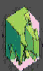Movies of Learning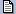Download Tech-Report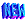Download the Software! The Decision Intersection Boundary Algorithm (DIBA) disambiguates the "distributed" representation of multiple hidden layer, multiple output threshold feed-forward neural networks. DIBA Generates a direct mapping between a network's inputs and outputs in the form of polytopic decision regions. That is, it finds what regions in the networks input space correspond to different outputs. These can be viewed directly, in lower dimensions , or analyzed using bounding rectangles and slicing in higher dimensions. We can even use the algorithm to watch networks during learning, seeing their decision regions and weights changing simultaneously. The algorithm works well for moderate sized networks (all the networks here took less than 10 seconds to analyze on a PII). In larger networks, we expect to run into exponential space, an inherent aspect of network complexity. You can now download the code, and try DIBA for yourself. NOTE: If you are looking for an applied method to analyze decision regions, check out this new paper. It describes Decision Region Connectivity Analysis (DRCA), a method to extract decision region structure, completely independently of the model type or dimensionality. Thus it can be used to efficiently analyze even very high-dimensional models with tens of thousands of parameters.
 Introduction Despite being prolific models, feed-forward neural networks have been ubiquitously criticized for having a low degree of comprehensibility. A neural network could be performing a task it was trained for perfectly, but to its users it remains a black-box. What has it learned about the data it was trained on? Is it generalizing in a consistent manner? For what inputs is the network untrustworthy? These are questions we would like answers to. Different approaches have been put to this problem such as Rule-Extraction, Contribution Analysis and Network Inversion. In our approach we try to generate exact representations of a network's function by analyzing the principles of its underlying computation. Only by analyzing networks exactly can we learn their real strengths and pitfalls: When they will generalize well, the relationship between size and complexity, the relationship between dimensionality and complexity, when will learning succeed and fail, etc. In this page we present an algorithm tthat extracts exact representations from feed-forward threshold neural networks in the form of polytopic decision regions. We demonstrate its use on different networks, and show what this extracted representation can tell us about a network's function. We then use the underlying principles that the algorithm is based on to calculate network and algorithmic complexity bounds as well as raise questions about learning in neural networks. Algorithm Principals The algorithm extracts polytopic decision regions from multi-layer multi-output feed-forward threshold activation function networks. The algorithm is based on a few observations on how these neural networks compute: Networks are deterministic, output is a direct function of input. The location where an output unit switches values is a Decision Boundary. This corresponds to a change in the output units' partial sum, which in turn corresponds to a change in the output of one or more hidden units. The locations of change are at the intersections of the first hidden layer's units' hyperplanes. The network divides up the input space into polytopic Decision Regions whos verticies are at the intersections of the hidden unit hyperplanes. Algorithm Logic The Decision Intersection Boundary Algorithm (DIBA) receives as inputs the weights of a three layer feed forward network and the bounds of the input space. Its output consists of the vertex pairs, or line segment edges that describe the decision regions in input space. The algorithm consists of two parts, a part which generates all the possible vertices and connecting line segments, and a part that evaluates whether these basic elements form the boundaries of decision regions. The generative part performs a recursion over the intersections of the hidden unit hyperplanes. This is achieved by incrementally projecting hyperplanes onto each other. A boundary in an n dimensional input space demarcates the location of an output value transition. For an intersection of hyperplanes to be a boundary we seek that all hyperplanes making up the intersection have at least one face that acts as a boundary in its vicinity. The same boundary test is used for vertices and edges. Circle Network Using back-propagation, an 80 hidden unit sigmoidal network was trained to classify points inside and outside of a circle of radius 1 around the origin. Treating the activation functions as threshold, the DIBA algorithm was used to generate the immediate figure showing the network's representation of the learnt circle-- a decision region composed of the hidden unit line boundaries. The decision region fully describes what the network is doing. That is, all points inside the decision region will generate a network output of 1, and all the points outside the decision region will generate a network output of 0. We can see that the network did a pretty good job of encapsulating a circle-like decision regions, but we can also see where the learned circle deviates from an actual circle and how some of the training points were misclassified.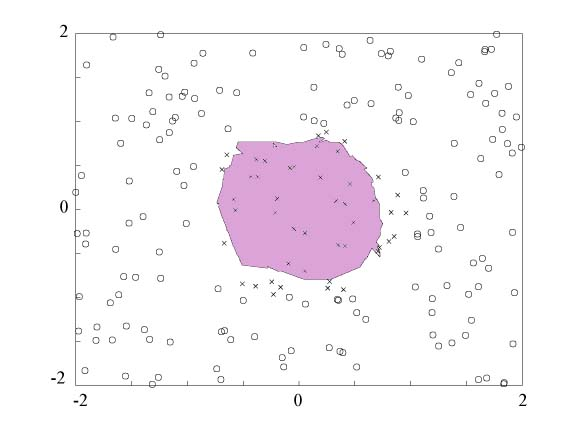By zooming out from the area in the immediate vicinity of the origin we can see the network's performance or generalization ability, in areas of the input space that it was not explicitly trained for. In the figure we see some artifacts, decision regions at a distance of at least 50 units from the decision region at the origin. This implies a network misclassification if that part of the input space was of interest.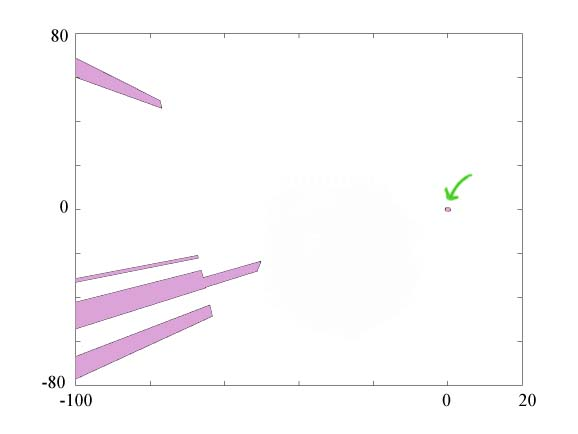Sphere Network A 100 hidden unit network was trained to differentiate between points inside and outside of a sphere centered at the origin. The figure below illustrates the network's sphere-like decision region from two angles. The figure below shows the same artifact phenomena as in the circle network above-- the miniature sphere appears amid a backdrop of an ominous cliff face decision region.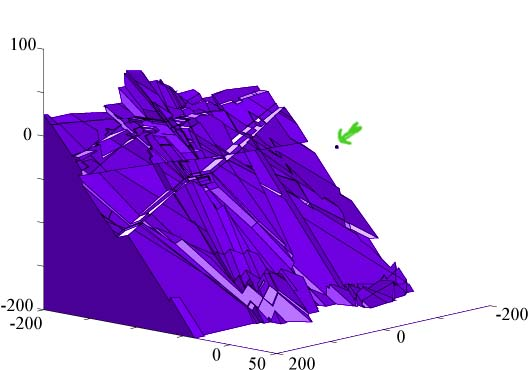4-D Sphere Network A 100 hidden unit network was trained to recognize points inside and outside of a four dimensional hyper-sphere centered around the origin. Since its dimensionality is greater than three it is not possible to directly visualize the decision region. One way to gather information from our high-dimensional polytopic decision regions is to describe them in terms of rules. That is, we bound each polytope inside a hyper-rectangle, and examine the rectangle's coordinates. The rectangles can later be refined to enclose parts of polytopes, thereby giving higher resolution rules. In this case our decision region is covered by the following hyper-rectangle: min: (-4.45,-5.79,-3.90,-7.93) max: ( 6.47, 6.01, 7.39, 6.07) Another way to examine the high-dimensional polytope is to examine projections on to lower dimensional spaces. In this figure we see two projections of the 4-D sphere decision region. Needless to say the 4-D sphere looks less and less like an actual sphere. It is fair to speculate that this is due to the difficulties of learning in higher dimensional spaces. The higher dimensionality naturally promotes more complex decision regions, with potential for greater artifact phenomena.Ball Throwing Network A 40 hidden unit network was trained to predict whether a thrown ball will hit a target. As input, it received the throwing angle, initial velocity and the target distance. It achieved an 87% success rate on its training data.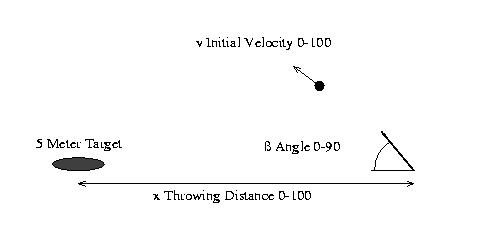In the following figure, the network's decision region is contrasted with the analytical solution to the problem. The network managed to encapsulate the gist of the analytical model, but it may miss a few jump shots.S&P 500 Direction Predictor Network A neural network with 40 hidden units was trained to predict the average direction of movement (up or down) for the following month's S&P 500 Index. It was trained on macro economic data from 1953 to 1994. The network's inputs are: the percentage change in the S&P 500 from the past month, the differential between the 10-year and 3-month Treasury Bill interest rates, and the corporate bond differential for AAA and BAA rates. After training, the network achieved a greater than 80% success rate on the data. In the figure we can see the network's decision regions. Immediately we can surmise that the network would probably not generalize very well to out of sample data. We can see that rather than extracting some underlying regularity, some nice localized geometrical description of the data points, the network tended to highly partition the space with small and irregular decision regions to try and enclose all the sporadic data points. In the region of input space where the training data resides the network has 28 different decision regions. Of these, all but five have a bounding rectangle with a volume of less than one. One decision region's rectangle encompasses the whole space. If we slice this polytope using three hyperplanes, each bisecting the input space across a different dimension, then if the polytope was completely convex we would expect, at most, to get eight sub polytopes. However for this decision region, the slicing generates 23 sub-polytopes, suggesting a high degree of concavity, possibly an irregular branching structure which highly partitions the input space.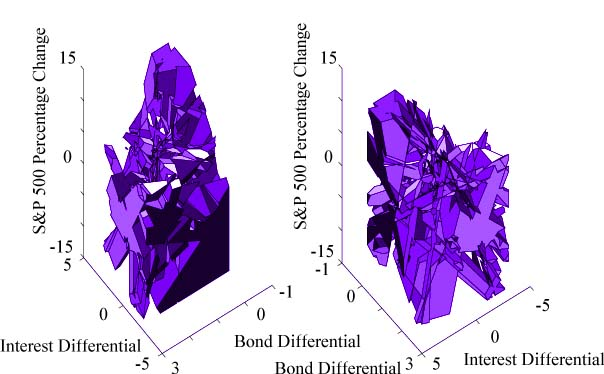Vowel Recognition Network Two neural networks were trained on vowel data available at the CMU repository. This data consists of 10 log-area parameters of the reflection coefficients of 11 different steady-state vowel sounds. Our interest in this example was to gauge the effect of using input spaces of different dimensionality. Whether adding dimensions will improve the generalization of the network. Both networks were trained to recognize the vowel with the had sound contrasted with another 10 vowel sounds. The first network received as input the first two coefficients. After training, it achieved a better than 86% success rate on the training data. The following figure illustrates its decision regions. In the input region of [3.2,2.3]X[0.7,1.2] we see that many disparate decision regions are used to secure a perfect classification.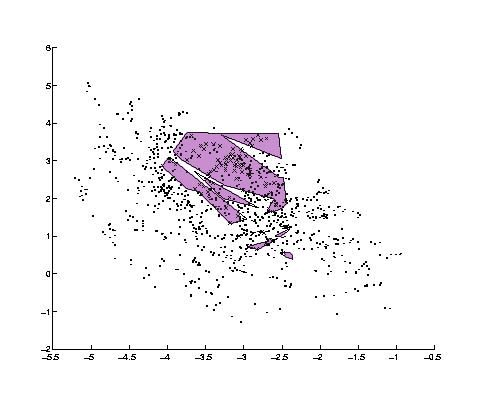The second network received the first four coefficients as inputs. It achieved a success rate of over 91% on the training data. It also achieved perfect classification in the same input region. However it doesn't seem to have partitioned the space. There is only one decision region in this area. If we slice this polytope using four hyperplanes bisecting each of its dimensions, we only get 10 sub polytopes, suggesting a small degree of concavity (less than the 16 we would expect for a perfectly convex shape). In addition these sub-decision regions are mostly delimited in the third and fourth dimension with the first two dimensions ignored (span the whole space). Therefore, it appears that the network makes use of these added dimensions to form a more regular decision region in that difficult portion of the input space. Algorithm and Network Complexity The Decision Intersection Boundary Algorithm's complexity stems from its transversal of the hyperplane arrangement in the first layer of hidden units. As such, that part of its complexity is equivalent to similar algorithms, such as arrangement construction [Edelsbrunner 87], which are exponential in the input dimension. Do we really need to examine every vertex though? Perhaps the network can only use a small number of decision regions, or it is limited with respect to the complexity of the decision regions. The following figure demonstrates that a network is capable of exponential complexity by an inductive construction of a network with an exponential number of decision regions, where each decision region has an exponential number of vertices. The figure shows the inductive step from one dimension to two dimensions. The zeros represent decision regions (line in 1-D, squares in 2-D).Generalization and Learning Generalization is the ability of the network to correctly classify points in the input space that it was not explicitly trained for. In a semi-parametric model like a neural network, generalization is the ability to describe the correct output for groups of points without explicitly accounting for each point individually. Thus, the model must employ some underlying mechanisms to classify a large number of points from a smaller set of parameters. In our feed-forward neural network model we can characterize the possible forms of generalization with two mechanisms. The first is by proximity: nearby points in the same decision region are classified the same way. The second is by face sharing: the same hidden unit hyperplane is used as a border face in either multiple decision regions or multiple times in a decision region. Given these two mechanisms, how well do learning algorithms exploit them? It is intuitive that learning algorithms which pull borders (Hyperplanes in this case) towards similar output points and away from different output points, should be geared for proximity generalization by forming decision regions. However face sharing is more combinatorial in nature, and might not be as amenable to continuous deformation of parameters as found in many algorithms. To illustrate this point a group of 8 hidden unit neural networks were trained on the decision regions illustrated in the 2-D complexity figure. One thousand data points were taken as a training sample. Two hundred different networks were tested. Each was initialized with random weights between -2 and 2, and trained for 300 online epochs of backpropagation with momentum. Of the 200 networks none managed to learn the desired 9 decision regions. The network with the best performance generated 6 decision regions (left figure) out of an initial two. However, in examining its output unit's weights, we see that only one weight changed sign, the weight initially closest to zero, indicating a predisposition to this configuration in the initial conditions. The other figures are the decision regions of the more successful networks.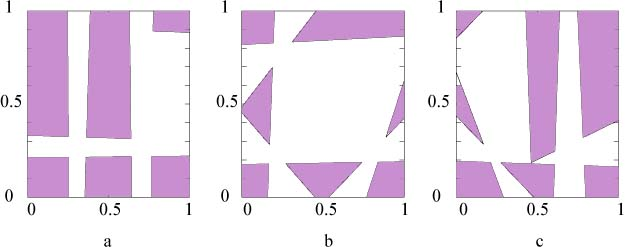Network Learning Animations If we can visualize the decision regions of a neural network, as is the case for networks with two or three inputs, then we can visualize these networks as they learn-- watching how their decision regions are modified with iterations of the learning algorithm. The following three movies are of networks trying to learn the decision region indicated by their respective picture. That is, a network trying to learn a sphere, a cylinder and three separate spherical decision regions. Each frame consists of the network's decision regions juxtaposed with a Hinton diagram of the network's weights. The blue cloumn has the output unit's weights, while the gray rows are the hidden unit weights. These movies are examples of relatively successful learning using backprop with momentum. Their successes are not the norm, and more frequently than not learning will result in inappropriate or non-existant decision regions. Note, even though we are interpreting the activation functions as threshold for the algorithm, backprop is treating them as sigmoids. The implications of this are outlined in the tech-report.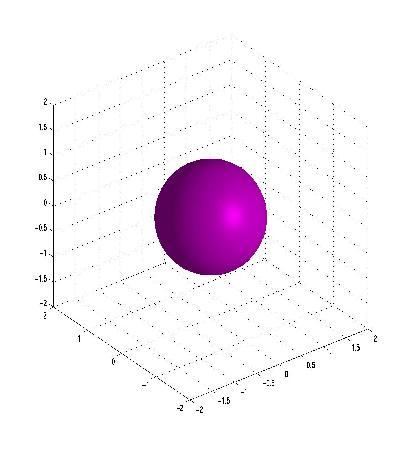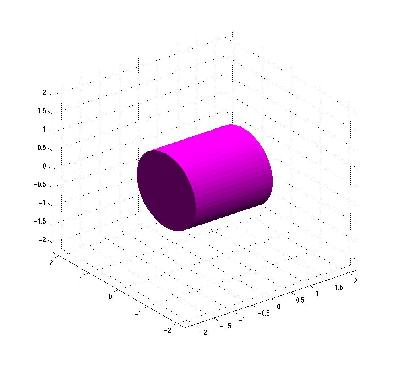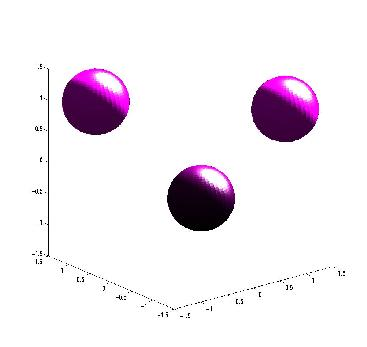Quicktime MPEG Quicktime MPEG Quicktime MPEG
 Software Download Now the basic DIBA algorithm is available as C++ source code under the Gnu Public License. It has been compiled under Linux, but should compile under any standard C++ compiler and libraries. The three dimensional output formats available are Matlab, Povray and MHD. I will not be providing support for this program. But if you generate something interesting using it, or find a novel application, let me know at melnik@cs.brandeis.edu, and I will add a link to your page here. The package can be download from here.